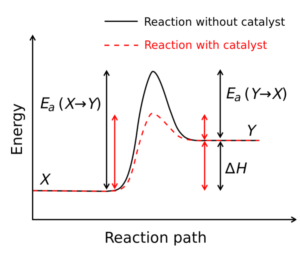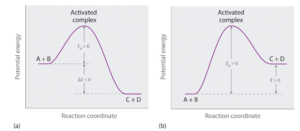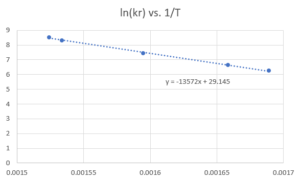# 16 Arrhenius Equation

Learning Objectives

By the end of this chapter, you should be able to:

Explain the effect of temperature on reaction rates

The Arrhenius Equation describes the relationship between the reaction rate and temperature.

 $ln(k_{r})=ln(A)-\frac{E_{a}}{RT}$

Where

• $A$ in units of $time^{-1}$ (eg. $s^{-1}$,$min^{-1}$…) is called the frequency factor or pre-exponential factor. It describes the frequency that molecules collide with the correct orientation to possibly make a chemical reaction.
• $E_{a}$ is the activation energy in units of energy per amount of molecules (eg.J/mol, kJ/mol…) This is introduced in more details later in this session.
• $R$ is the ideal gas constant in the appropriate units (eg. 8.314$\frac{J}{mol·K}$)
• $T$ needs to be expressed in absolute units (eg. K)

The Arrhenius Equation is in linear form if we graph $ln(k_{r})$ as y and $\frac{1}{T}$ as x:

 $ln(k_{r})=-\frac{E_{a}}{R}×\frac{1}{T}+ln(A)$

Where

• The slope of the graph is equal to $-\frac{E_{a}}{R}$
• The y-intercept is equal to $ln(A)$

Once $E_{a}$ is known for a reaction, the rate of reaction can be predicted at a given temperature if it is known at another temperature:

$ln(k_{r1})=ln(A)-\frac{E_{a}}{RT_{1}}$ and $ln(k_{r2})=ln(A)-\frac{E_{a}}{RT_{2}}$

Subtract the first from the second to get:

$ln(k_{r2})-ln(k_{r1})=-\frac{E_{a}}{RT_{2}}+\frac{E_{a}}{RT_{1}}$

This simplifies to:

 $ln\Big(\frac{k_{r2}}{k_{r1}}\Big)=\frac{E_{a}}{R}\Big(\frac{1}{T_{1}}-\frac{1}{T_{2}}\Big)$

Exercise: Reaction Rate Change with Changing Temperature

Say a certain biological reaction has an activation energy of 50 kJ/mol, how much faster will this reaction be at body temperature (37°C) vs room temperature (25°C)?

Note R = 8.314$\frac{J}{mol·K}$

### Solution

• Be careful with the unit consistency. Use absolute units for temperatures.

$ln\Big(\frac{k_{r2}}{k_{r1}}\Big)=\frac{50×10^3J/mol}{8.314J/(mol·K)}\Big(\frac{1}{298K}-\frac{1}{310K}\Big)=0.781$

$\frac{k_{r2}}{k_{r1}}=e^{0.781}=2.18$

So with this 12°C temperature increase, the reaction rate would increase 2.18 times. A rule of thumb is that reaction rates double every 10°C, but we know this depends on the activation energy.

Manipulating the previous Arrhenius equation, we can isolate $k_{r}$ by taking the exponent of each side to get:

$k_{r}=Ae^{(-\frac{E_{a}}{RT})}$

If Ea is 0 (all collisions result in a reaction) then:

$k_{r}=Ae^{(-\frac{E_{a}}{RT})}=Ae^0=A$

Meaning the rate constant when each collision is successful is equal to the frequency factor.

When $Ea>0$, then only a subset of reactions are successful represented by $e^{(-\frac{E_{a}}{RT})}$

Catalysts speed up the reactions usually by decreasing the activation energy. Sometimes catalysts can affect frequency factors as well, but the effect of frequency factor is minor in comparison to the decrease of activation energy. This is demonstrated in the example question after the activation energy session.Image from Wikimedia Commons

Exercise: Effect of Temperature on Equilibrium

1.  True or False: A reaction is in a state of equilibrium when the equilibrium constant K is equal to 0.$^{}$

2. Which of the following does not affect the concentration of each component at equilibrium for a reaction system?$^{}$

b. Increasing temperature

c. Using a catalyst

d. Decreasing volume

Hint: An understanding of Le Châtelier’s principle can be helpful for this question, but you can also analyze this question using the definition of $K_{eq}$

• Le Chatelier’s principle: this principle states that temperature, volume, concentration, or pressure changes result in equilibrium shifts that re-establish a new equilibrium state.

### Solution

1. False– because for each specific reaction, the equilibrium constant only depends on temperature. The reaction is at equilibrium when the value of $\prod_{i} a_{i}^{vi}$ is equal to $K_{eq}$ (notice $a_{i}$ instead of $a_{i,eq}$, because we don’t know if the system is at equilibrium when we calculate this). Also experimentally, we can say that the system is at equilibrium when the concentrations of each component are not changing.

Using a catalyst decreases the energy barrier needed for the reaction to proceed (activation energy), but does not affect the equilibrium constant.

Both a and d will cause shifting of equilibrium due to Le Chatelier’s principle. For a, the reaction will shift to the reverse, meaning that the reverse reaction will happen more often so more reactants will be formed and more products will be consumed. For d, the system will shift to the side that produces a larger number of gaseous molecules to increase the volume. There may be a case where gas volume stays the same no matter with products or reactants, but this is not known.

If you analyze a and d using $K_{eq}$, the equilibrium constant will not change, but the concentration of the components will change, and the system will approach the same equilibrium constant after the change.

b – The equilibrium constant will change when temperature changes, shifting reactant and product concentrations.

Activation Energy

The activation energy($E_{a}$) represents the energy needed for the reactants to collide and get to a state such that their bonds can rearrange to form the products.

$ln(\frac{k_{r2}}{k_{r1}})=\frac{E_{a}}{R}(\frac{1}{T_{1}}-\frac{1}{T_{2}})$

• A high $E_{a}$ indicates the rate strongly depends on the temperature
• Zero $E_{a}$ indicates the rate is independent of temperature
• Negative $E_{a}$ indicates the rate decreases with temperature (this is very rare)

Graph (a) illustrates the general situation in which the products have lower potential energy than the reactants, so the overall reaction releases energy; that is, it is energetically downhill, and ΔE < 0.

In contrast, graph (b) illustrates the case in which the products have higher potential energy than the reactants, so the overall reaction requires an input of energy; that is, it is energetically uphill, and ΔE > 0.

Although the energy changes that result from a reaction can be positive, negative, or even zero, in most cases an energy barrier must be overcome before a reaction can occur. This means that the activation energy is almost always positive.$^{}$Image from Chemistry Libretexts / CC BY-NC-SA 3.0

Example: Catalyst Affecting Activation Energy

Enzymes are biological catalysts. Suppose we have a reaction with an activation energy of 80 kJ and a frequency factor of 1 without catalysts at 298K, calculate the reaction rate constant without catalyst and compare the rate constant (by taking the ratio of k) if the enzyme:

a) Decreases the activation energy to 8kJ.

b) Increases the frequency factor to 10.

Solution

$k$ without catalyst:
\begin{align*}
k(uncatalyzed)& =Ae^{(-\frac{E_{a}}{RT})}\\
& = 1×e^{(-\frac{80×10^3J}{8.314J/(molK)298K})}\\
& = 9.48×10^{-15}
\end{align*}

a)
\begin{align*}
\frac{k'(catalyzed)}{k(uncatalyzed)} & =\frac{Ae^{(-\frac{E_{a}’}{RT})}}{Ae^{(-\frac{E_{a}}{RT})}}\\
& = e^{\frac{-E_{a}’+E_{a}}{RT}}\\
& = e^{\frac{(-8+80)×10^3J/mol}{8.314J/(molK)298K}}\\
& = 4.2×10^{12}
\end{align*}

b)
\begin{align*}
\frac{k'(catalyzed)}{k(uncatalyzed)} & =\frac{A’e^{(-\frac{E_{a}}{RT})}}{Ae^{(-\frac{E_{a}}{RT})}}\\
& = \frac{A’}{A}\\
& = 10
\end{align*}

A decrease in $E_{a}$ has a drastic effect on reaction rate.

Exercise: Activation Energy Calculation

The equation for the decomposition of $NO_{2}$ to $NO$ and $O_{2}$ is second order in $NO_{2}$:

$2NO_{2(g)}→2NO_{(g)}+O_{2(g)}$

Data for the reaction rate as a function of temperature are listed in the following table. Calculate Ea for the reaction and the rate constant at 700 K.$^{}$

 T(K) k $(M^{-1}s^{-1})$ 592 522 603 755 627 1700 652 4020 656 5030

### Solution

Step 1: Plot the Arrhenius Equation in the linear form: $ln(k_{r})=-\frac{E_{a}}{R}*\frac{1}{T}+ln(A)$Step 2: Calculate the activation energy ($E_{a}$) and the frequency factor ($A$) from the slope and intercept\begin{align*}
E_{a} & = -slope × R\\
& = -(-13572 K)×8.314 J/(molK)\\
& = 112838 J/mol\\
& = 112.8 kJ/mol
\end{align*}\begin{align*}
A & = e^{y-intercept}\\
& = e^{29.145}\\
& = 4.545×10^{12} M^{-1}s^{-1}
\end{align*}Note that the units of A are the same as kr, but we tend to then remove the units when we take logarithm as we usually consider products of logarithmic. The important thing to remember is if we use A to solve for kr (as we do below), we need to include the relevant units.Step 3: Calculate the rate constant ($k_{r}$) using the $E_{a}$, $A$ and given temperature

\begin{align*}
k_{r} & =Ae^{(-\frac{E_{a}}{RT})} \\
& = 4.545×10^{12}e^{(-\frac{112838 J/mol}{8.314 J/(molK)×700 K})} \\
& = 17265 M^{-1}s^{-1}\\
& = 1.73 × 10^{4} M^{-1}s^{-1}
\end{align*}

## References

 Chemistry LibreTexts. 2020. Characteristics of the Equilibrium State.

 Chemistry LibreTexts. 2019. 4.6 Activation Energy and Rate. [online] Available at: <https://chem.libretexts.org/Courses/Bellarmine_University/BU%3A_Chem_104_(Christianson)/Phase_2%3A_Chemical_Kinetics/4%3A_Kinetics%3A_How_Fast_Reactions_Go/4.6%3A_Activation_Energy_and_Rate> [Accessed 24 April, 2020].